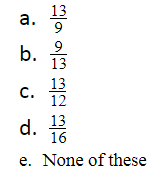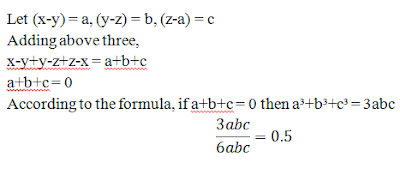New Students Offer - Use Code HELLO

# Simplification Questions For IBPS RRB Mains 2017Ques 1. If p = 168, then find the value ofa. 168
b. 130
c. 169
d. 13
e. None of these

Ques 2. If A = 11, B =13, C = 5 and P:Q = 2:7, R:S = 2:7, T:U= 2:7 then find the value ofa. 1 : 1
b. 1 : 2
c. 2 : 7
d. Cannot be determined
e. None of these

Ques 3. If A(A+B+C) = 9, B(A+B+C) = 16, C(A+B+C) = 144 then the value of A will beQues 4. If x = 167, y = 519 thena. 1
b. 2
c. 0
d. 4
e. None of these

Ques 5. 1)   If the value of x is 119 solvea. Cannot be determined
b. 0
c. 1
d. 119
e. 118/119

Ques 6. Solve the following.a. 0
b. 1
c. 0.5
d. Cannot be determined
e. 2

1. 169

#### Solutions2. 2:7

#### Solutions

Trick:
a:b = c:d = e:f = n:k then
Thenwhere n, k, u, v, w are any integer number.

3. 9/13

#### Solutions4. 2

#### Solutions

Trick:
According to the formula, this value is always equal to 2.5. 119

#### Solution

Trick:
According to the formula, this value is always equal to x.6. 0.5

#### Solution